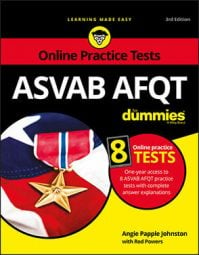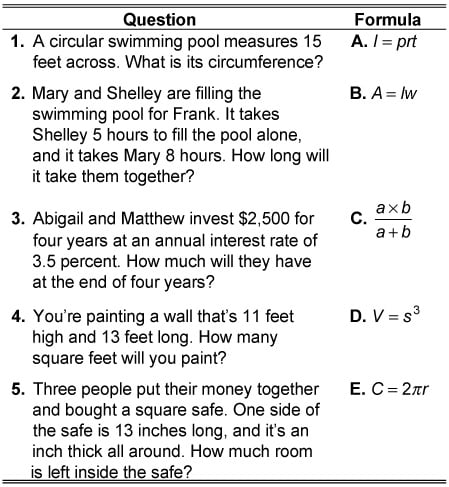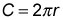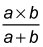##### ASVAB AFQT For DummiesSome math problems on the ASVAB AFQT will require you to apply formulas to solve real-world problems. It's important to not only be familiar with these formulas, but also to know when and how to apply them.

## Practice exercise

In the following practice exercise, you need to match the word problem to the appropriate formula. (You don't actually need to solve the problems—this exercise is just to test your ability to choose the right formula.) When you're finished, check the "Answers and explanations" that follow.1. The correct answer is Choice E.The problem asks you to find the circumference of a circle, and the formula for that iswhere C represents circumference and r represents the circle's radius. Remember, the radius of a circle is half its diameter.

2. The correct answer is Choice C.The formula to solve a work problem that asks you how long it will take two people together to accomplish a task is3. The correct answer is Choice A.Investment and loan problems can typically be solved with the interest formula, which is I = prt. I stands for interest, p represents the principal, r represents the interest rate, and t represents the amount of time you're evaluating.
4. The correct answer is Choice B.When a problem asks you, "How many square feet … ," it's looking for an area. The formula for the area of a rectangle is A = lw, where A represents area, l represents length, and w represents width.
5. The correct answer is Choice D.In this problem, you need to use the formula for the volume of a cube, which is V = s3, where V represents volume and s represents the length of a side.